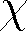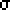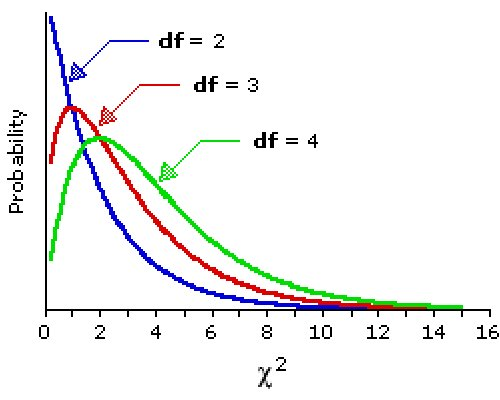# The Chi Square Frequency Test

#### Lesson Overview

The test statistics used in conjunction with the normal and Student t distributions assume certain parameters about the parent populations, specifically, normality and variance homogeneity. Quite often in behavioral science research such restrictive assumptions cannot be made and certain nonparametric tests have been developed which help us analyze such data. A common distribution encountered in such nonparametric tests is the2 distribution.

### Chi Square Distributions and Tests

The2 distribution is a continuous distribution related to the normal distribution. Specifically it involves the sum of squares of normally distributed random variables. Chi is a Greek letter () and is pronounced like the hard k sound in the Scottish work Loch (and not like those grassy chia pets). The2 distribution is important in several contexts, most commonly involving variance.

The2 family of distributions is characterized by one parameter called the degrees of freedom which is often denoted by v (the greek letter nu) and used as a subscript:2v.

1. The2 distribution is continuous.
2. The2 distribution is unimodal.
3. The2 distribution is always positive (>0).
4. The2 distribution mean = v.
5. The2 distribution variance = 2v.
6. For small v (v < 10), the distribution is highly skewed to the right (positive).
7. As v increases the2 distribution becomes more symmetrical about v (the mean).
8. We can thus approximate the2v when v > 30 with the normal (see table below).
Tables of critical2 values are commonly available (as below) or can be computed by a statistical package or statistical calculator.

Gosset first described the distribution of s2. It is related to the2 by the simple factor (n-1)/2. Although he wasn't able to prove this mathematically, he demonstrated it by dividing a prison population of 3000 into 750 random samples of size four and used their heights.

A common application of the2 distribution is in the comparison of expected with observed frequencies. When there is but one nominal variable, this is often termed goodness of fit. In this case we are testing whether or not the observed frequencies are within statistical fluctuations of the expected frequencies. Although one typically checks for high2 values, the second example below illustrates the possible significance of a low2 value.

Example: On July 14, 2005 we collected 10 trials of 20 pennies each where these 20 pennies were set on edge and the table banged. We observed 145 heads. We can compare the observed with expected frequencies and test for goodness of fit as shown in the table below. There is but one degree of freedom since the number of tails is dependent on the number of heads (200 - 145 = 55).

Side: Head 145 55 100 100 45 -45 2045 2045 20.45 20.45

Solution: We form the2 statistic by summing the (O-E)2/E and get 2045/100 + 2045/100 = 40.9. We can then compare this2 with critical2 values or find an associated P-value. The critical2 value for df=1 and one-tailed, alpha=0.05 is 3.841. Our results are far to the right of 3.841 so are VERY significant (P-value=1.6×10-10). A table of critical2 values for select values is given below.

### A Chi Square Distribution Table

df\upper tail area0.990.950.900.100.050.01
10.000160.00390.0162.7063.8416.635
20.020 0.103 0.2114.6055.9919.210
30.115 0.352 0.5846.2517.81511.34
40.297 0.711 1.0647.7799.48813.28
50.554 1.145 1.6109.23611.0715.09
102.558 3.940 4.86515.9918.3123.21
155.229 7.261 8.54722.3125.0030.58
208.260 10.85 12.4428.4131.4137.57
2511.52 14.61 16.4734.3837.6544.31
df > 30: use z = sqrt(2chi2)-sqrt(2df-1)Example: On July 12, 2005 we collected 192 dice rolls, each person present using a different die and each person doing 24 rolls. Were the results within the expected range?

Pips: 1 2 3 4 5 27 23 30 35 40 37 32 32 32 32 32 32 -5 -9 -2 3 8 5 25 81 4 9 64 25 0.78125 2.53125 0.125 0.28125 2.00 0.78125

Solution: We form the2 statistic by summing the (O-E)2/E and get 208/32=6.5. We can then compare this2 with a critical2. Only if it is more extreme is it worth finding a P-value. We have 6 - 1 = 5 degrees of freedom. The critical2 values for df=5, two-tailed, and alpha=0.10 are 1.145 and 11.07. Since our2 is within this range, our results are within the range we can expect to occur by chance. Notice the lower2 cut off. When people fabricate a random distribution they are likely to make it too uniform and get too small of a2 which can be checked as above, but the2 would likely be less than 1.145. Working backwards we see the sum of the (O-E)2 would have to be less than 36 so if one were 5 or less away and the rest much closer, we might wonder.

As noted at the bottom of the table above, when the degrees of freedom are large, a z-score can be formed and compared against a standard normal distribution. Note also that the mean of any2 is the degrees of freedom. This might be helpful to realize where the distribution is centered.

The2 goodness of fit does not indicate what specifically is signficant. To find that out one must calculate the standardized residuals. The standardized residual is the signed square root of each category's contribution to the2 or R = (O - E)/sqrt(E). When a standardized residual has a magnitude greater than 2.00, the corresponding category is considered a major contributor to the significance. (It might be just as easy to see which (O - E)2/E entries are larger than 4, but standardized residuals are typically provided by software packages.)

### Other Applications

The2 goodness of fit test can be extended to more than one variable. It then is often termed the2 test of homogeneity. Contingency tables are formed, expected frequencies are derived from the marginal totals, the2 computed, and checked. The degrees of freedom will be (R - 1)(C - 1), where R is the number of rows and C is the number of columns. The null hypothesis is that there is no difference in variable one between variable two. Similar tests can be performed when the null hypothesis is stated somewhat differently (no relationship, form phi, test OR the proportion in state one of variable one is the same as the proportion in state two of variable one, form proportion difference, test).

There are potential problems associated with small expected frequencies in contingency tables. Historically, when any cell of a 2×2 table was less than 5 a Yates' correction of continuity was advised. However, it has been shown that this can result in a loss of power (a tendancy not to reject a false null hypothesis). Care should be exercised and advise sought. Larger contingency tables can also be problematic when more than 20% of the cells have expected frequencies less than 5 of if there are any cells with 0. One solution is to combine adjacent rows or columns, but only if it makes sense.

The McNemar test is a2 test for matched pair (like pre-/post-test) treatment designs. In the 2×2 contingency table, the A and D cells contain the change responses and the B and C cells contain the no change responses. The2 simplifies to (A - D)2/(A + D) and is interpretted as per usual (with df = 1).

The Stuart-Maxwell test extends the McNemar test to 3×3 contingency tables. Here the no change situation occupies the main diagonal (upper left to lower right) and we form the2 from averaged pairs of differences weighted by the square of the differences between the other row/column totals. We leave the curious reader to a software package or statistics textbook for the actual formula.

Remember, the prior lesson referred to the Pearson contingency coefficient (C) and Cramer's V coefficient which are defined in terms of the2 statistic. Specifically, C = sqrt(chi2/(n + chi2)) and V = sqrt(chi2/(n(q -1 )), where q is the smaller of the number of rows or columns in the contingency table.

In closing we should note the importance of focusing on a small number of well-conceived hypotheses in research rather than blindly calculating a bevy of2 statistics for all variable pairs and ending up with 5% of your results being significant at the 0.05 level! You would even expect 1% of your results, due to pure random chance in your sample selection, to be significant at the 0.01 level. Since there are n(n - 1)/2 possible pairings for n variables, one would have 5050 pairs for 100 variables of which over 250 could look significant at the 0.05 level. Beware!